CAT  >  Banker's Discount - Examples (with Solutions)

# Banker's Discount - Examples (with Solutions) Notes | Study Logical Reasoning (LR) and Data Interpretation (DI) - CAT

## Document Description: Banker's Discount - Examples (with Solutions) for CAT 2022 is part of Logical Reasoning (LR) and Data Interpretation (DI) preparation. The notes and questions for Banker's Discount - Examples (with Solutions) have been prepared according to the CAT exam syllabus. Information about Banker's Discount - Examples (with Solutions) covers topics like and Banker's Discount - Examples (with Solutions) Example, for CAT 2022 Exam. Find important definitions, questions, notes, meanings, examples, exercises and tests below for Banker's Discount - Examples (with Solutions).

Introduction of Banker's Discount - Examples (with Solutions) in English is available as part of our Logical Reasoning (LR) and Data Interpretation (DI) for CAT & Banker's Discount - Examples (with Solutions) in Hindi for Logical Reasoning (LR) and Data Interpretation (DI) course. Download more important topics related with notes, lectures and mock test series for CAT Exam by signing up for free. CAT: Banker's Discount - Examples (with Solutions) Notes | Study Logical Reasoning (LR) and Data Interpretation (DI) - CAT
 1 Crore+ students have signed up on EduRev. Have you?

Question 1: The banker's gain on a sum due 3 years hence at 12% per annum is Rs.270.The banker's discount is:
(1) 920
(2) 480
(3) 1020
(4) 640
Option 3
Explanation:

T.D.=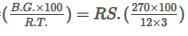= Rs.750
∴ B.D.= Rs.(750+270) = Rs.1020

Question 2: The banker's discount on Rs.1600 at 15% per annum is the same as ture discount on Rs.1680 for the same time and at the same rate. The time is :
(1) 2 months
(2) 4 months
(3) 6 months
(4) 1 months
Option 2
Explanation:
S.I. on Rs.1600=T.D.on  Rs.1680.
∴ Rs.1600 is the P.W of Rs 1680,i.e Rs 80 is S.I. on Rs 1600 at 15%
∴ Time = (100×80/1600×15) year = 1/3 year = 4 months.

Question 3: The banker's discount on a sum of money for 112 years is Rs.558 and the true discount on the same sum for 2 years is Rs.600.The rate percent is :
(1) 10%
(2) 14%
(3) 12%
(4) 18%
Option 3
Explanation:
B.D. for 3/2 years.= Rs.558 B.D for 2 years = Rs.(558×2/3×2) = Rs.774
T.D. for 2 years =Rs.600.
∴ sum = (B.D.×T.D/B.D.−T.D) = Rs (744×600/144) = Rs.3100
Thus,Rs 744 is S.I on Rs 3100 for 2 years.
∴ Rate = (100×744/3100×2) % = 12%

Question 4: The banker's discount of a certain sum of money is Rs.72 and the true discount on the same sum for the same time is Rs.60.The sum due is :
(1) Rs. 360
(2) Rs. 342
(3) Rs. 558
(4) RS.1056
Option 1
Explanation:
Sum = B.D×T.D/B.D.−T.D. = (72×60/72−60) = (72×60/12) = Rs 360.

Question 5: The true discount on a bill or Rs 540 is Rs 90.The banker's discount is :
(1) 50
(2) 108
(3) 120
(4) 142
Option 2
Explanation:
P.W = Rs.(540-90) = Rs.450.
∴ S.I on Rs 450 = Rs 90
S.I on Rs 540 = Rs (90/450×540)= Rs 108.
∴ B.D = Rs 108.

Question 6: The present worth of a certain bill due sometime hence is Rs.800 and the true discount is Rs.36.The banker's discount is :
(1) 27
(2) 37.62
(3) 24.63
(4) 25
Option 2
Explanation:
B.G = T.D2/P.W=Rs.(36×36/800) = Rs 1.62
∴ B.D = (T.D + B.G)= Rs (36 + 1.62) = Rs 37.62

Question 7: The banker's gain on a certain sum due 112 years hence is 325 of the banker's discount .The rate percent is :
(1) 5(1/5)
(2) 9(1/11)
(3) 4(2/5)
(4) 3(1/5)
Option 2
Explanation:
Let, B.D = Rs 1
Then B.G = Rs 3/25
∴ T.D = (B.D - B.G) = Rs(1−3/25)=Rs 22/25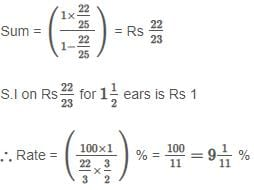Question 8: The banker's discount on a certain sum due 2 years hence is 1110 of the  true discount .The rate percent is :
(1) 10%
(2) 12%
(3) 5%
(4) 2.5%
Option 3
Explanation:
Let T.D. be  Rs.1 .Then,B.D.=Rs.11/10= Rs.1.10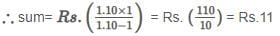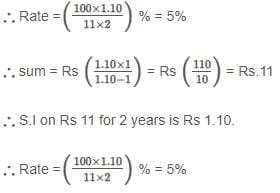Question 9: The banker's gain on a bill due 1 year hence at 12% per  annum is Rs.6. The hence discount is :
(1) 62
(2) 47
(3) 24
(4) 50
Option 4
Explanation:
T.D.=B.G.×100/R×T = Rs(6×100/12×1) = Rs.50

Question 10: The banker's discount on a bill due 4 months hence at 15% is Rs.420.The true discount is :
(1) 400
(2) 385
(3) 580
(4) 220
Option 1
Explanation: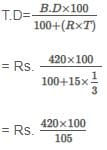= Rs. 400

Question 11: The present worth of a sum due sometime hence is Rs.576 and the banker's  gain is Rs.16.The true discount is :
(1) 26
(2) 62
(3) 28
(4) 96
Option 4
Explanation:
T.D.=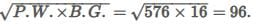Question 12: The present worth of a certain sum due sometime hence is Rs.1600 and the true discount is Rs.160. The banker's gain is :
(1) 10
(2) 26
(3) 16
(4) 21
Option 3
Explanation:
B.G. = T.D.2/P.W. = Rs.(160×160/1600) = Rs.16

Question 13: The banker's gain of a certain sum due 2 years hence at 10% per annum is Rs.24.The present worth is :
(1) Rs.350
(2) Rs.420
(3) Rs. 600
(4) 863
Option 3
Explanation: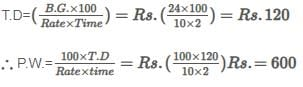The document Banker's Discount - Examples (with Solutions) Notes | Study Logical Reasoning (LR) and Data Interpretation (DI) - CAT is a part of the CAT Course Logical Reasoning (LR) and Data Interpretation (DI).
All you need of CAT at this link: CAT

## Logical Reasoning (LR) and Data Interpretation (DI)

96 videos|90 docs|119 tests
 Use Code STAYHOME200 and get INR 200 additional OFF

## Logical Reasoning (LR) and Data Interpretation (DI)

96 videos|90 docs|119 tests

### How to Prepare for CAT

Read our guide to prepare for CAT which is created by Toppers & the best Teachers

Track your progress, build streaks, highlight & save important lessons and more!

,

,

,

,

,

,

,

,

,

,

,

,

,

,

,

,

,

,

,

,

,

;